## MTSO December News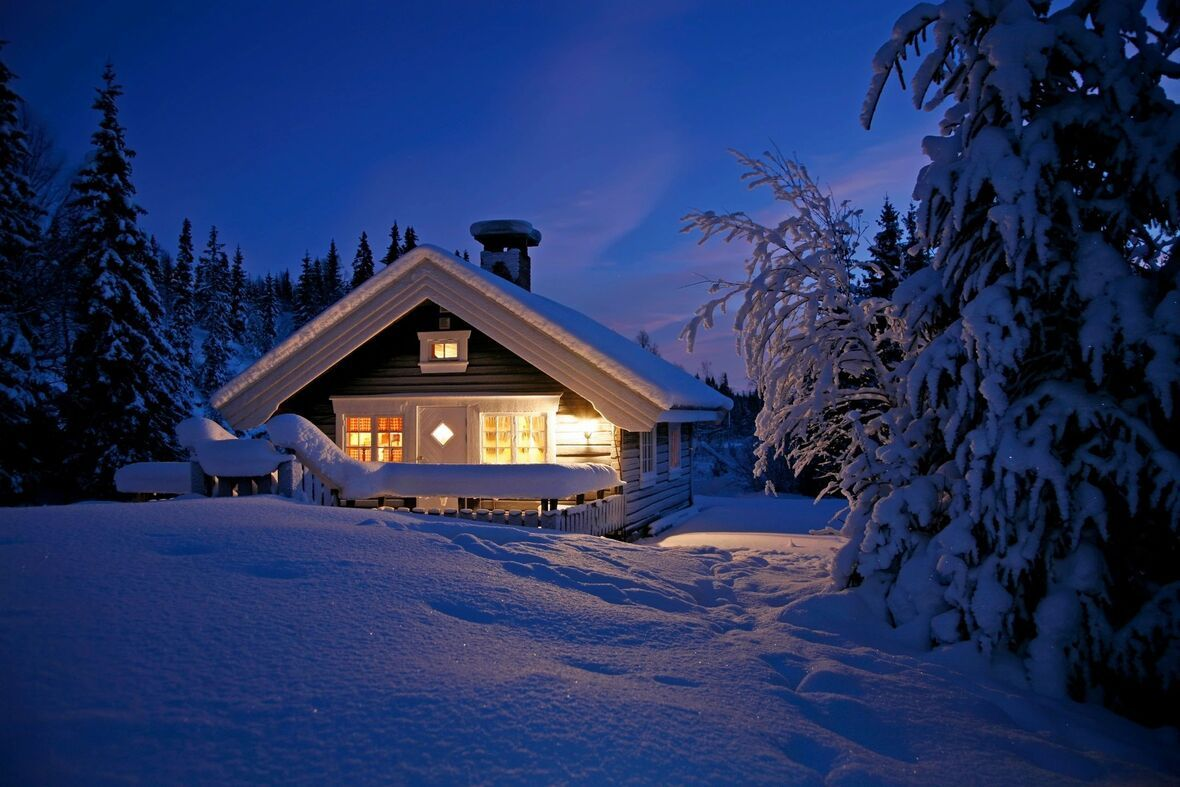We hope that you, and your family are staying healthy and safe this season. We all have had to maneuver around the impacts of this pandemic and the unprecedented resulting effects. We extend our sincere thanks to our loyal customers for your ongoing support and loyal dedication during this challenging time. We are all in this together, and we will get through it together. Stay safe, be well and focus on health. - MTSO Staff

 table div table+table+table+table div table{width:100%;padding:0}table div table+table+table+table div table img{width:96.23%;padding:0;float:none}table div table+table+table+table div table td{width:100%;padding:0 1.88% 18px}/* styles */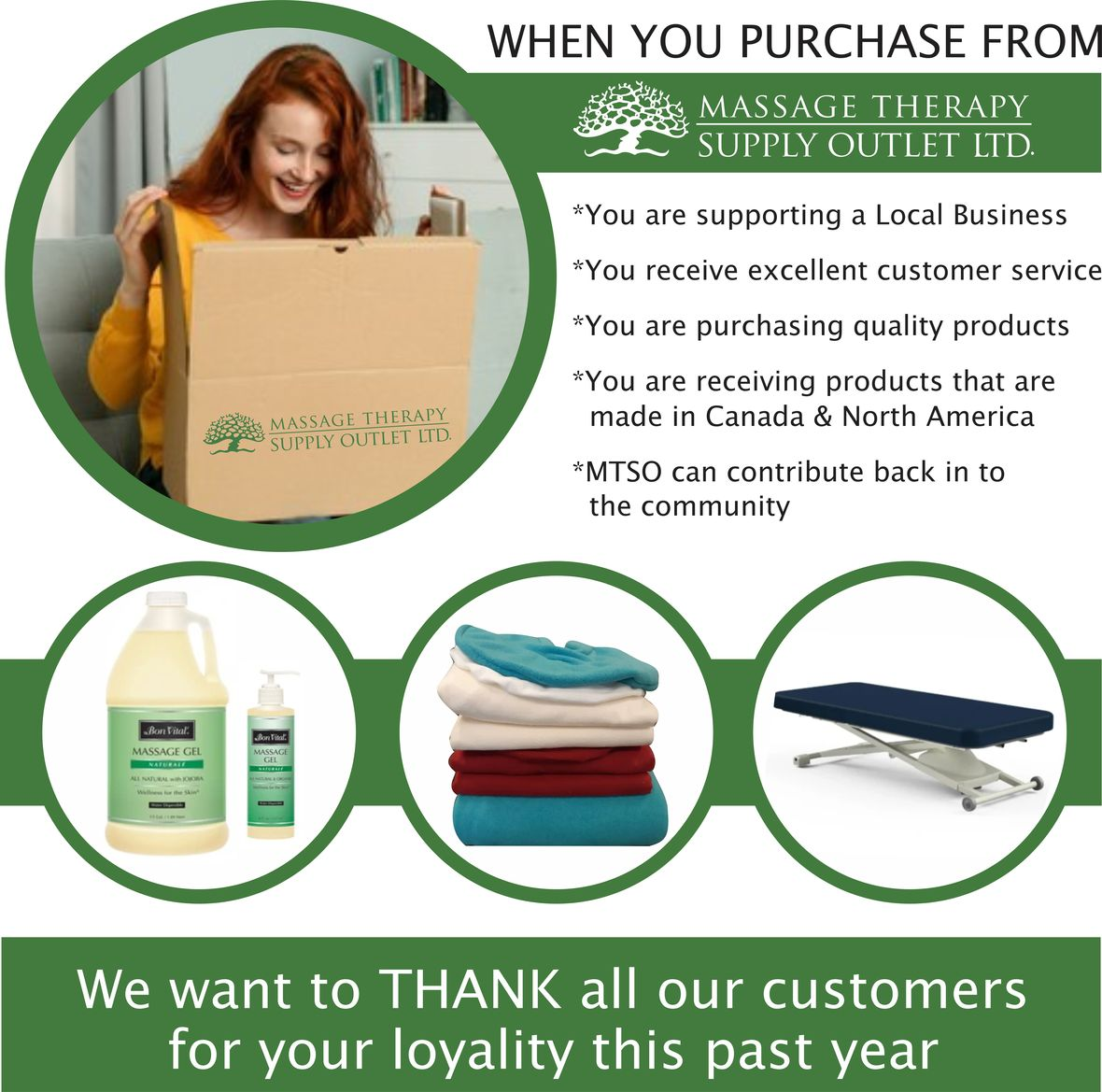# CURBSIDE PICKUP IS AVAILABLE

In addition to in-store shopping and shipping, curbside pick-up is also available. You can either phone-in your order or place order online. When placing your order online choose Local Pick Up at check out. We will contact you when your order is ready.

# REVISED STORE HOURS

Monday to Saturday 11:00AM - 4:00PM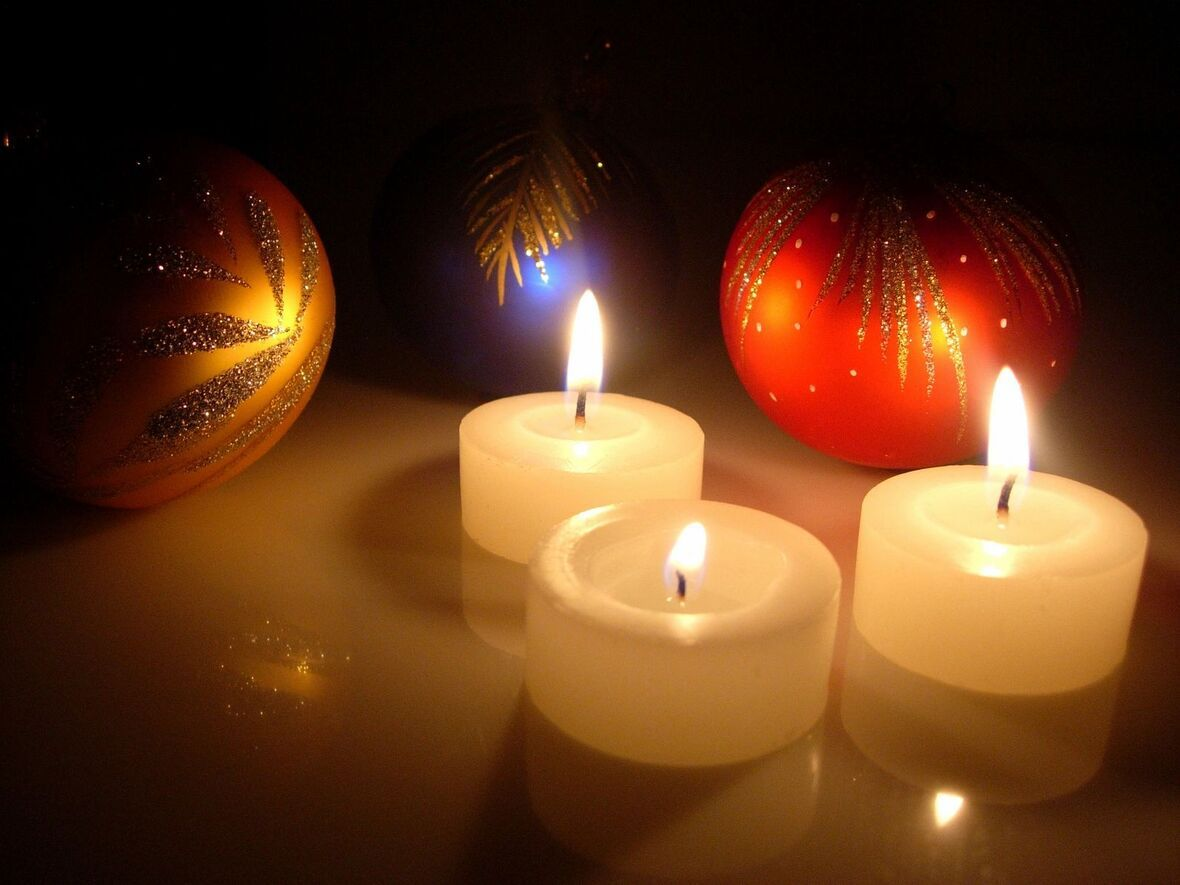# HOLIDAY HOURS

December 21-23: 11:00 AM - 4:00 PM
December 24-27: Closed
December 28-30: 11:00 AM - 4:00 PM
December 31 - January 1: Closed
January 2: 11:00 AM - 4:00 PM

 table.module-9{width:83.02%;padding:0}table div table+table+table+table+table+table+table+table+table+table div table{width:83.02%;float:none;margin-left:auto;margin-right:auto;padding:0}table div table+table+table+table+table+table+table+table+table+table div table a{border:0 none;text-decoration:none}table div table+table+table+table+table+table+table+table+table+table div table img{width:100%!important;border:0 none;text-decoration:none}table div table+table+table+table+table+table+table+table+table+table div table td{width:100%;padding:0}/* styles */

# Price: \$1799.00

▪ The Earthlite Ellora electric lift table is designed for comfort, easy care and reliability.
▪ Pro-Plush™ Deluxe 3-Layer 3” Cushion with rounded corners.
▪ Hands-free foot control and wheels for ease of movement.
 ▪ The Earthlite Ellora electric lift table is designed for comfort, easy care and reliability.
 ▪ Pro-Plush™ Deluxe 3-Layer 3” Cushion with rounded corners.
 ▪ Hands-free foot control and wheels for ease of movement.
 table div table+table+table+table+table+table+table+table+table+table+table+table+table div table{width:100%;padding:0}table div table+table+table+table+table+table+table+table+table+table+table+table+table div table img{width:96.23%;padding:0;float:none}table div table+table+table+table+table+table+table+table+table+table+table+table+table div table td{width:100%;padding:0 1.88% 18px}/* styles */## Holiday Aromatherapy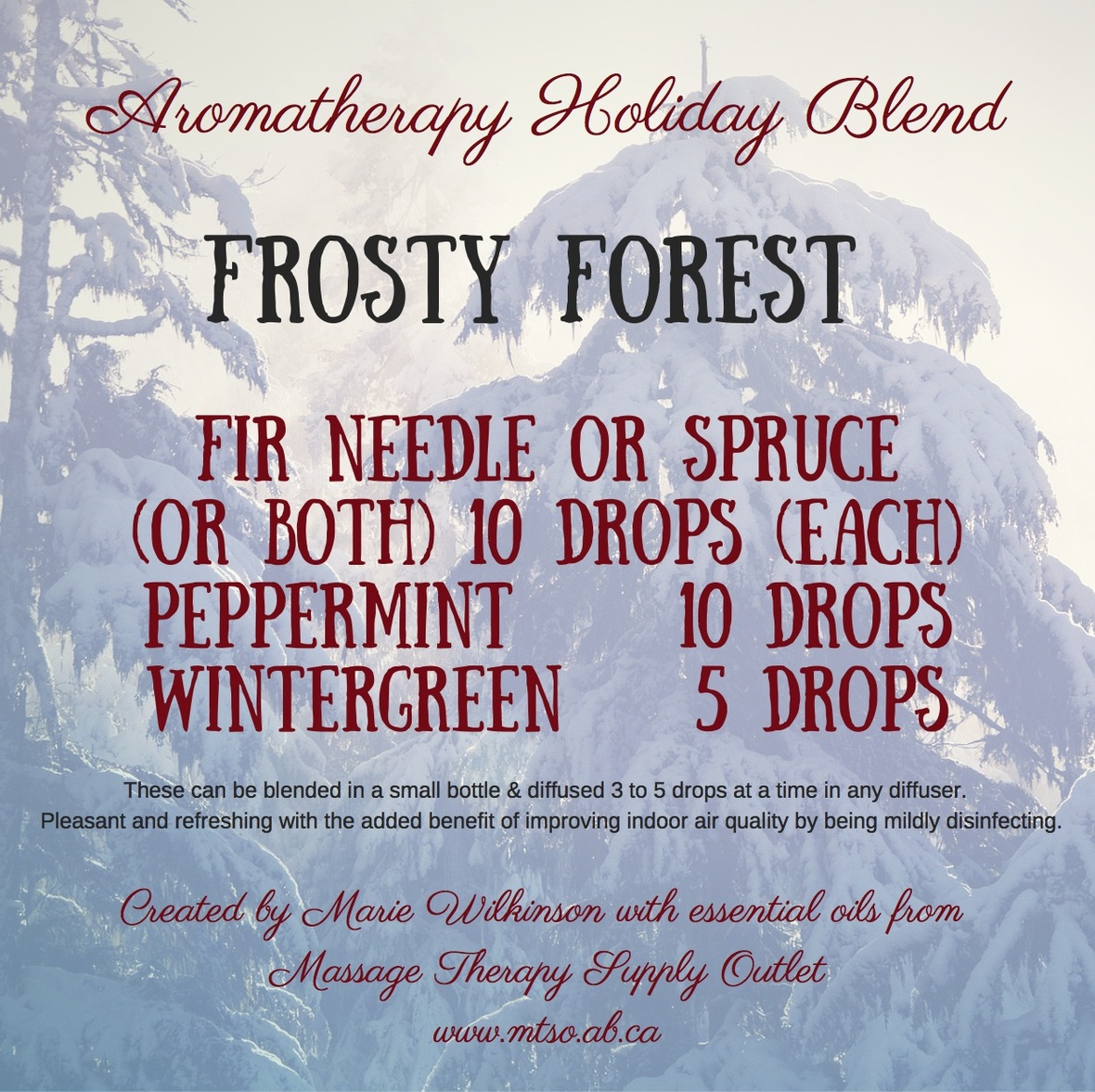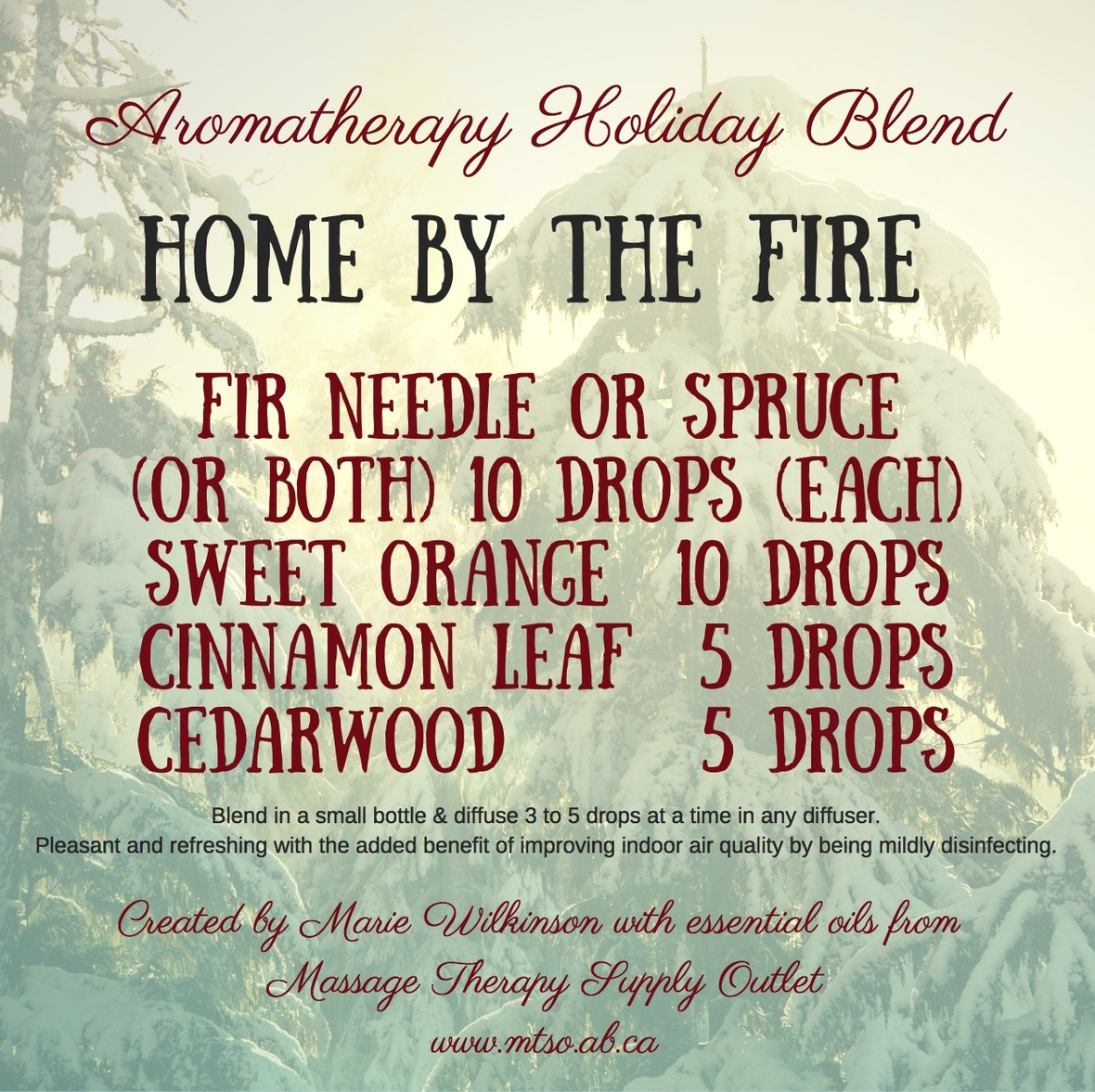## Our favourite seasonal blends

You'll love the beneficial effects of any of these delightful holiday blends - transform your space with nature's aromatic essences.

Link HERE to our essential oils for all the ingredients.

 table div table+table+table+table+table+table+table+table+table+table+table+table+table+table+table+table+table div table{width:100%;padding:0}table div table+table+table+table+table+table+table+table+table+table+table+table+table+table+table+table+table div table img{width:96.23%;padding:0;float:none}table div table+table+table+table+table+table+table+table+table+table+table+table+table+table+table+table+table div table td{width:100%;padding:0 1.88% 18px}/* styles */table.module-17{width:89.06%;padding:0}table div table+table+table+table+table+table+table+table+table+table+table+table+table+table+table+table+table+table div table{width:89.06%;float:none;margin-left:auto;margin-right:auto;padding:0}table div table+table+table+table+table+table+table+table+table+table+table+table+table+table+table+table+table+table div table a{border:0 none;text-decoration:none}table div table+table+table+table+table+table+table+table+table+table+table+table+table+table+table+table+table+table div table img{width:100%!important;border:0 none;text-decoration:none}table div table+table+table+table+table+table+table+table+table+table+table+table+table+table+table+table+table+table div table td{width:100%;padding:0}/* styles */
 table div table+table+table+table+table+table+table+table+table+table+table+table+table+table+table+table+table+table+table+table div table{width:100%;padding:0}table div table+table+table+table+table+table+table+table+table+table+table+table+table+table+table+table+table+table+table+table div table img{width:96.23%;padding:0;float:none}table div table+table+table+table+table+table+table+table+table+table+table+table+table+table+table+table+table+table+table+table div table td{width:100%;padding:0 1.88% 18px}/* styles */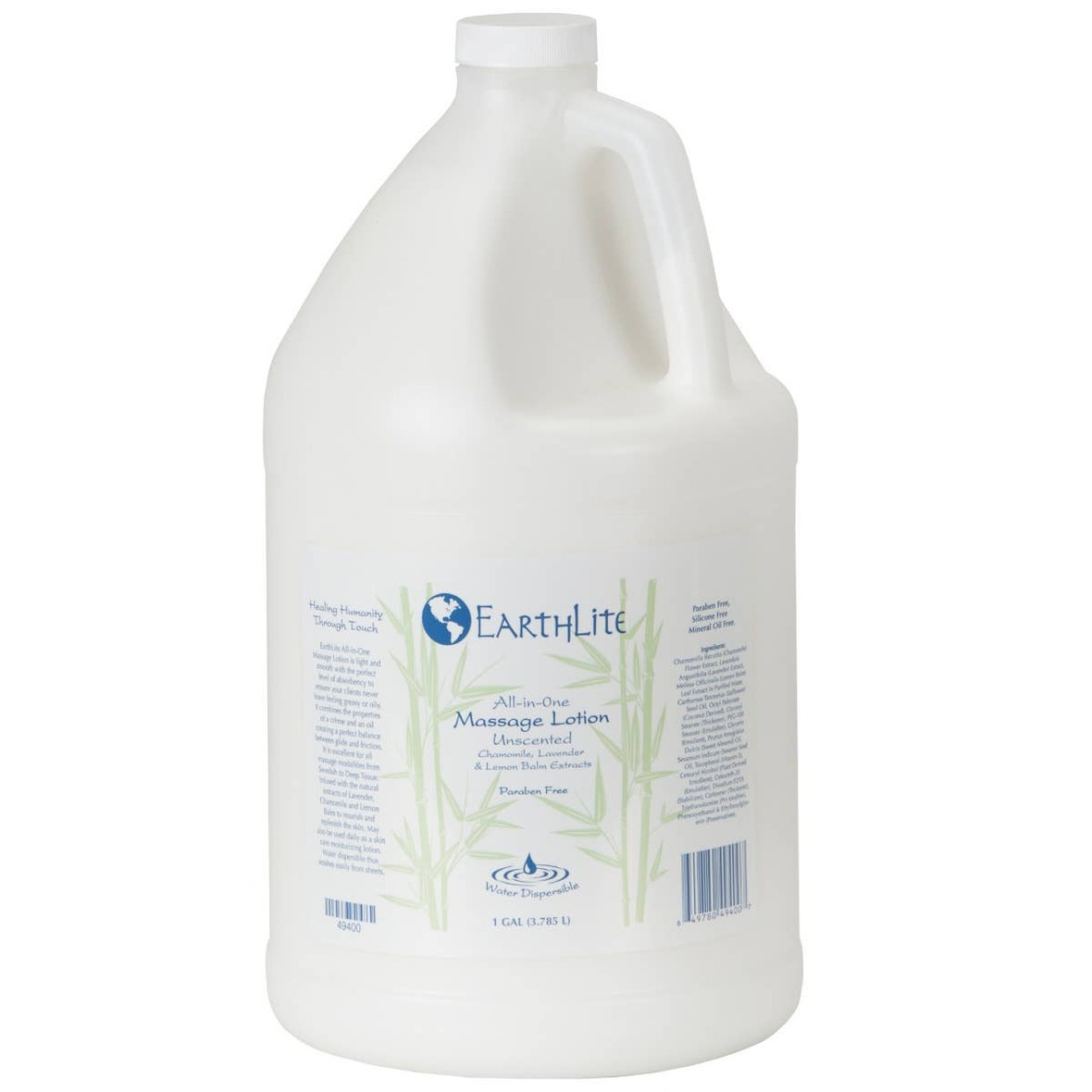Earthlite All-In-One Massage Lotion 1 Gallon

# *NEW Earthlite All-In-One Massage Lotion 1 Gallon

This Lotion is light and smooth with the perfect level of absorbency to ensure your clients never leave feeling greasy or oily.

It combines the properties of a crème and an oil having a perfect balance between glide and friction. Paraben Free, Silicone Free and Mineral Oil Free

 table div table+table+table+table+table+table+table+table+table+table+table+table+table+table+table+table+table+table+table+table+table+table+table div table{width:100%;padding:0}table div table+table+table+table+table+table+table+table+table+table+table+table+table+table+table+table+table+table+table+table+table+table+table div table img{width:96.23%;padding:0;float:none}table div table+table+table+table+table+table+table+table+table+table+table+table+table+table+table+table+table+table+table+table+table+table+table div table td{width:100%;padding:0 1.88% 18px}/* styles */# *NEW Earthlite Professional Table Warmer

This commercial grade Professional Massage Table Warmer and fleece pad in-one is made with a 0.5" cover and a 3-level temperature warming element with overheat protection for both exceptional comfort and safety.

 table div table+table+table+table+table+table+table+table+table+table+table+table+table+table+table+table+table+table+table+table+table+table+table+table+table+table div table{width:100%;padding:0}table div table+table+table+table+table+table+table+table+table+table+table+table+table+table+table+table+table+table+table+table+table+table+table+table+table+table div table img{width:96.23%;padding:0;float:none}table div table+table+table+table+table+table+table+table+table+table+table+table+table+table+table+table+table+table+table+table+table+table+table+table+table+table div table td{width:100%;padding:0 1.88% 18px}/* styles */## Stay in by the fire, order online for delivery or pickup

Browse and buy from an extensive collection at our online store, always open, 24/7/365. Edmonton store and showroom: 11:00 - 4:00 Mon-Sat.

 table.module-28{width:51.32%;padding:0}table div table+table+table+table+table+table+table+table+table+table+table+table+table+table+table+table+table+table+table+table+table+table+table+table+table+table+table+table+table div table{width:51.32%;float:none;margin-left:auto;margin-right:auto;padding:0}table div table+table+table+table+table+table+table+table+table+table+table+table+table+table+table+table+table+table+table+table+table+table+table+table+table+table+table+table+table div table a{border:0 none;text-decoration:none}table div table+table+table+table+table+table+table+table+table+table+table+table+table+table+table+table+table+table+table+table+table+table+table+table+table+table+table+table+table div table img{width:100%!important;border:0 none;text-decoration:none}table div table+table+table+table+table+table+table+table+table+table+table+table+table+table+table+table+table+table+table+table+table+table+table+table+table+table+table+table+table div table td{width:100%;padding:0}/* styles */
 Like   Tweet   Pin   in